# Electronics and Communication Engineering - Electromagnetic Field Theory

21.

A (75 - j 40) W load is connected to a co-axial line of z0 = 75ω at 6 MHz. The load matching on the line can be accomplished by connecting

 A. a short circuit stub at the load B. an inductance at the load C. a short circuit stub at a specific distance from the load D. a capacitance at a specific distance from the load

Answer: Option B

Explanation:

ZL = 75 - J . 40, ZC = 75 W, f = 6 mHz

Given ZL is capacitive, so proper matching, load must be inductive.

22.

A plane wave is characterized by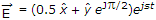the wave is

 A. linearly polarized B. circularly polarized C. elliptically polarized D. unpolarized

Answer: Option C

Explanation:

Because, magnitude is different (0.5, 1) and phase is 90°

23.

The electric field on the surface of a perfect conductor is 2V/m. The conductor is immersed in water with ε = 80 ε0. The surface charge density on the conductor is ((ε0 = 10-9/36p)F/m)

 A. 0 c/m2 B. 2 c/m2 C. 1.8 x 10-11 c/m2 D. 1.14 x 10-9 c/m2

Answer: Option D

Explanation: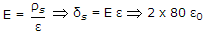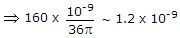.

24.

A wave is propagated in a waveguide at frequency of 9 GHz and separation is 2 cm between walls calculate the phase velocity for dominant mode.

 A. 3 x 108 m/sec B. 5 x 108 m/sec C. 1.5 x 108 m/sec D. 9 x 108 m/sec

Answer: Option B

Explanation:

Vg . Vp = C2

where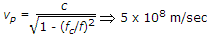.

25.

A plane electromagnetic wave travels in dielectric medium of relative permittivity 9. Relative to free space, the velocity of propagation in the dielectric is

 A. increased by a factor of 9 B. increased by a factor of 3 C. unchanged D. reduced by a factor of 1/3

Answer: Option D

Explanation: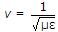.

#### Current Affairs 2021

Interview Questions and Answers